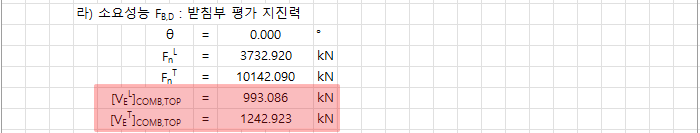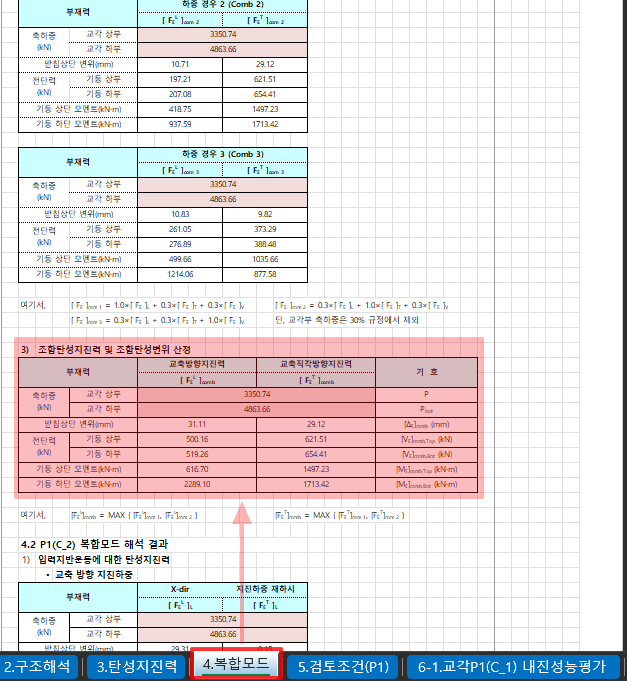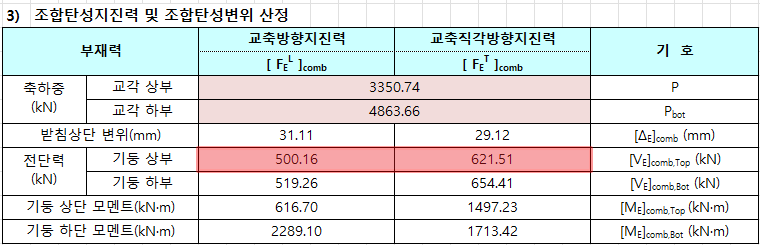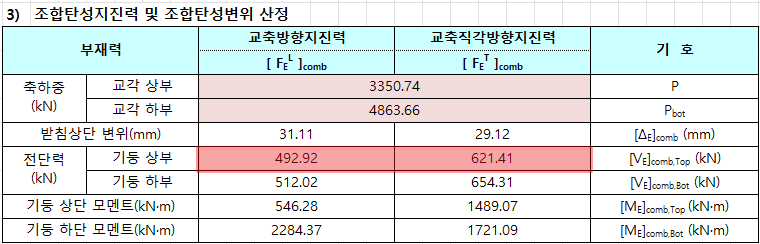Civil Created Edited

# [Civil] Calculation of the Combined Elastic Seismic Force used in Support Evaluation

## Question

How are the combined elastic seismic forces in each direction used for the required performance of the support evaluation calculated?The combined elastic seismic force used for the support evaluation is determined as the sum of the shear forces occurring at the top of the bridge pier columns and is organized into a table based on the results of member forces occurring at the top of the bridge pier columns in the "4. Composite Modes" sheet.The combined elastic seismic forces displayed on the "Support Seismic Performance Evaluation" sheet for each direction can be calculated as the sum of the shear forces occurring at the top of the bridge pier columns. In the example provided in the calculation sheet, where the bridge consists of two columns, the shear forces occurring at both columns are summed up as follows:

1. Results Table for Column P1_C1

Shear force at the top of the column: : 500.16kN(Horizontal) / 621.51kN(Vertical)2. Results Table for Column P1_C2

Shear force at the top of the column: : 492.92kN(Horizontal) / 621.41kN(Vertical)By summing up the shear forces occurring at the top of both columns for each direction, you can determine the combined elastic seismic forces used for the support evaluation:

Horizontal = 500.16 + 492.92 = 993.08kN

Vertical = 621.51 + 621.41 = 1242.92kN0